# KS1 Maths Quiz - Year 1 Fractions - Halves (Questions)

This quiz addresses the requirements of the National Curriculum KS1 Maths and Numeracy for children aged 5 and 6 in year 1. Specifically this quiz is aimed at the section dealing with recognising, finding and naming a half as one of two equal parts of an object, shape or quantity. It gently introduces them to fractions.

Halving numbers or groups of objects is often young children’s first experience of fractions. They may relate this to cutting one object in two, dividing by two or sharing one group into two equal parts. Children should recognise that for halving to be accurate, both parts should be equal in size or amount.

1.[ ] Cut it into 4 slices [ ] Cut it into 2 equal parts [ ] Share it with some friends [ ] Eat some quickly
2.[ ] Cut some out [ ] Share them into two equal groups [ ] Put them into 4 piles [ ] Put them in a bag
3.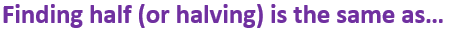[ ] Subtracting 2 [ ] Adding 2 [ ] Multiplying by 2 [ ] Dividing by 2
4.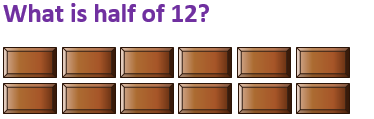[ ] 6 [ ] 12 [ ] 14 [ ] 24
5.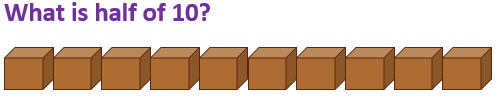[ ] 8 [ ] 5 [ ] 20 [ ] 35
6.[ ] 16 [ ] 14 [ ] 22 [ ] 10
7.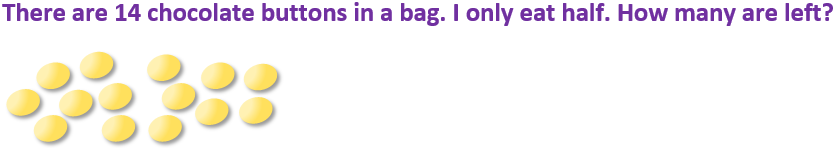[ ] 7 [ ] 6 [ ] 5 [ ] 10
8.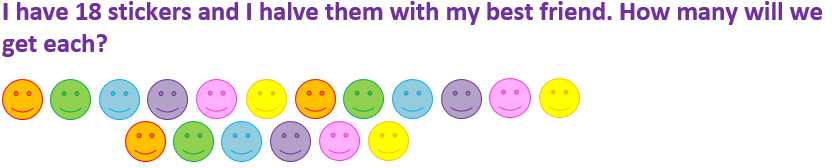[ ] 14 [ ] 10 [ ] 16 [ ] 9
9.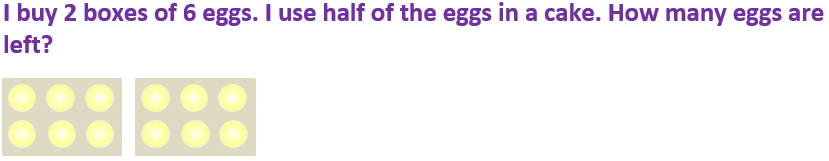[ ] 2 [ ] 16 [ ] 6 [ ] 4
10.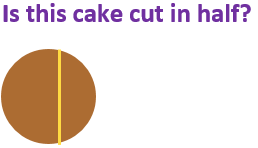[ ] Yes, there is a line down the middle [ ] Yes, it has been shared between two people [ ] Yes, there are two pieces [ ] No, the two pieces aren’t the same sizeKS1 Maths Quiz - Year 1 Fractions - Halves (Answers)
1.[ ] Cut it into 4 slices [x] Cut it into 2 equal parts [ ] Share it with some friends [ ] Eat some quickly
The two halves should be exactly the same size
2.[ ] Cut some out [x] Share them into two equal groups [ ] Put them into 4 piles [ ] Put them in a bag
Each group should have exactly the same number of stars
3.[ ] Subtracting 2 [ ] Adding 2 [ ] Multiplying by 2 [x] Dividing by 2
Halving means sharing into 2 equal groups or parts
4.[x] 6 [ ] 12 [ ] 14 [ ] 24
12 ÷ 2 = 6, so half of 12 is 6
5.[ ] 8 [x] 5 [ ] 20 [ ] 35
10 shared into 2 equal groups gives 5 in each group, so half of 10 is 5
6.[ ] 16 [ ] 14 [ ] 22 [x] 10
Sharing 20 into two equal groups would give 10 in each group
7.[x] 7 [ ] 6 [ ] 5 [ ] 10
Half of 14 is 7
8.[ ] 14 [ ] 10 [ ] 16 [x] 9
Half of 10 is 5, half of 8 is 4. 5 + 4 = 9
9.[ ] 2 [ ] 16 [x] 6 [ ] 4
Using half of the eggs would be the same as using one box only
10.[ ] Yes, there is a line down the middle [ ] Yes, it has been shared between two people [ ] Yes, there are two pieces [x] No, the two pieces aren’t the same size
Halves of a shape must be identical